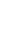# EMI Full Form - What is EMI?

Updated:21 Sep, 2022

EMI Stands for Equated Monthly Payment.

An equated monthly installment (EMI) is a fixed monthly payment made by a borrower to a creditor on a predetermined date. Each month, EMI is composed of a principal sum and an interest amount that a borrower must pay back over a set number of years in order to fully repay the loan. EMI is calculated using the loan amount, loan term, and interest rate charged by the bank on the loan.

## Advantages of EMI:

• By allowing people to pay in installments, EMI allows them to purchase items that are out of their financial reach.
• EMI has no effect on savings because they are required to pay minimum monthly payments rather than a lump sum.
• There is no hassle because the EMI is paid directly to the lender.

## Factors On Which EMI Depends:

The EMI depends on many factors, including

• Rate of Interest
• Principal amount borrowed
• Tenure of the loan

### Rate of Interest -

Every bank charges a set interest rate on the loan you take out. As a result, the Interest Rate is also required in order to calculate the exact amount of EMI. Because of the high-interest rate, high EMIs are calculated.

### Amount Borrowed(Loan amount) -

The loan amount is the amount of money you want to borrow from a bank. As a result, when calculating the Equated Monthly Instalment, you must include the required loan amount. The principal amount has the ability to increase or decrease your EMI. If you take out a large loan, your EMI will be high as well.

### Tenure -

You will also need to provide the loan tenure when calculating the EMI. If you want to pay a low EMI, extend the loan term, but this will increase the total amount of interest on your loan.

## Methods To Calculate EMI:

Flat Rate: When the loan is repaid in installments, each interest charge is calculated using the original principal amount in the flat rate method. The EMI is calculated by adding the total loan principal and interest to the principal, then dividing the total by the number of EMI installments, which is the time remaining on the loan term. On personal loans and auto loans, the flat rate strategy is popular. Borrowers benefit less from this strategy because interest payments must cover the entire principal amount, resulting in a higher effective interest rate than the reducing-balance strategy. Short-term loans, such as vehicle and motorcycle loans, typically have a Flat rate of interest.

Reducing Balance: In contrast to the flat rate method, the reducing balance strategy determines the interest payment based on the outstanding principal. This means that the interest and principal payment components of each EMI change. As a percentage of the outstanding loan, interest payments make up a higher amount of the EMI at the start of the loan period. As the loan is repaid over time, the interest amount reduces and a greater proportion of the payments go toward principal repayments. On house mortgages, credit cards, and overdraft services, the reducing-balance method is frequently used.